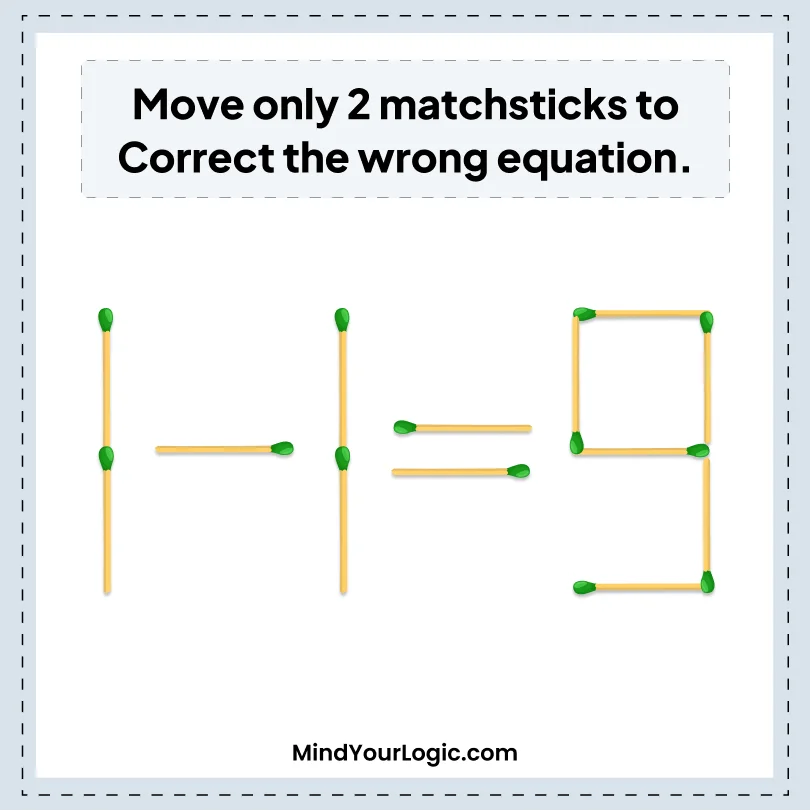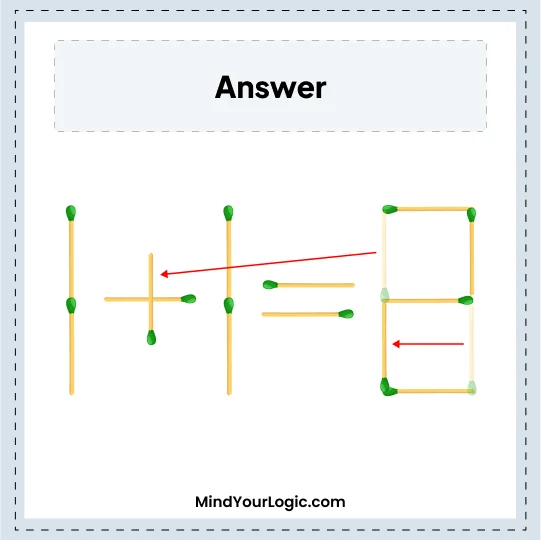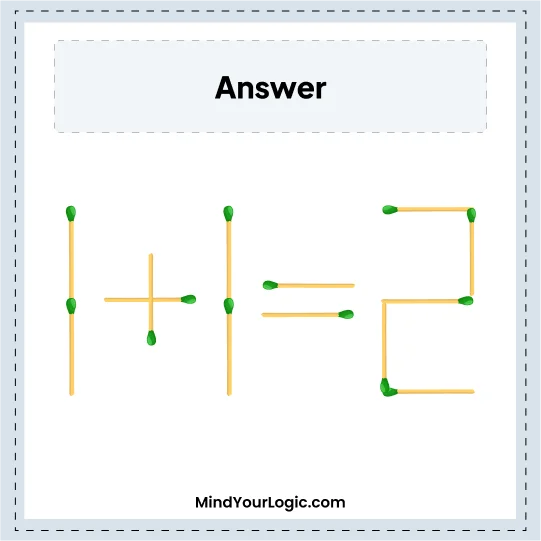# 1-1 = 9 - Matchstick Puzzles

###### 9.Matchstick Puzzles
`Move only 2 matchsticks to correct the wrong equation.`•

Explanation :

``` Move one stick from 9 to + sign, which becomes +,
again move one stick forward from 9, which becomes 2,
the correct equation we get is 1 + 1 = 2. ```